Back

## Sum in the Circle

In a circle puzzle like the ones below, dashed arrows mean to add and solid arrows mean to multiply. For example, the solution to the puzzle on the left is a number whose sum is $5 + 9,$ which is $14.$ The solution to the puzzle on the right is a number that, when multiplied by $6,$ gives us $12.$ By working backwards, we get a solution of $2.$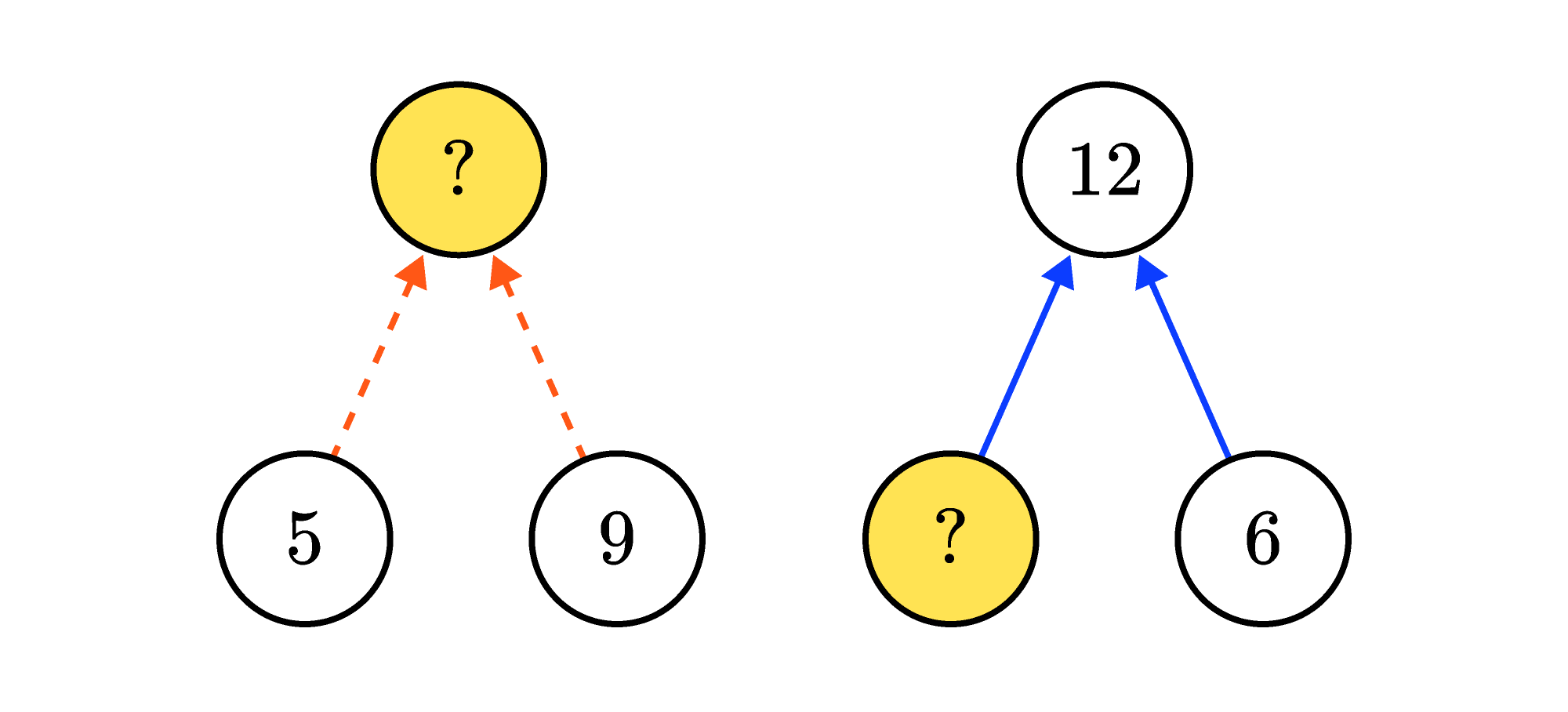Keep reading to see a few more complicated example puzzles, or dive right into today's challenge if you feel ready.

The two circle puzzles we just saw are both one-step puzzles. To solve multi-step circle puzzles, we might need to use multiple arithmetic steps in the correct order.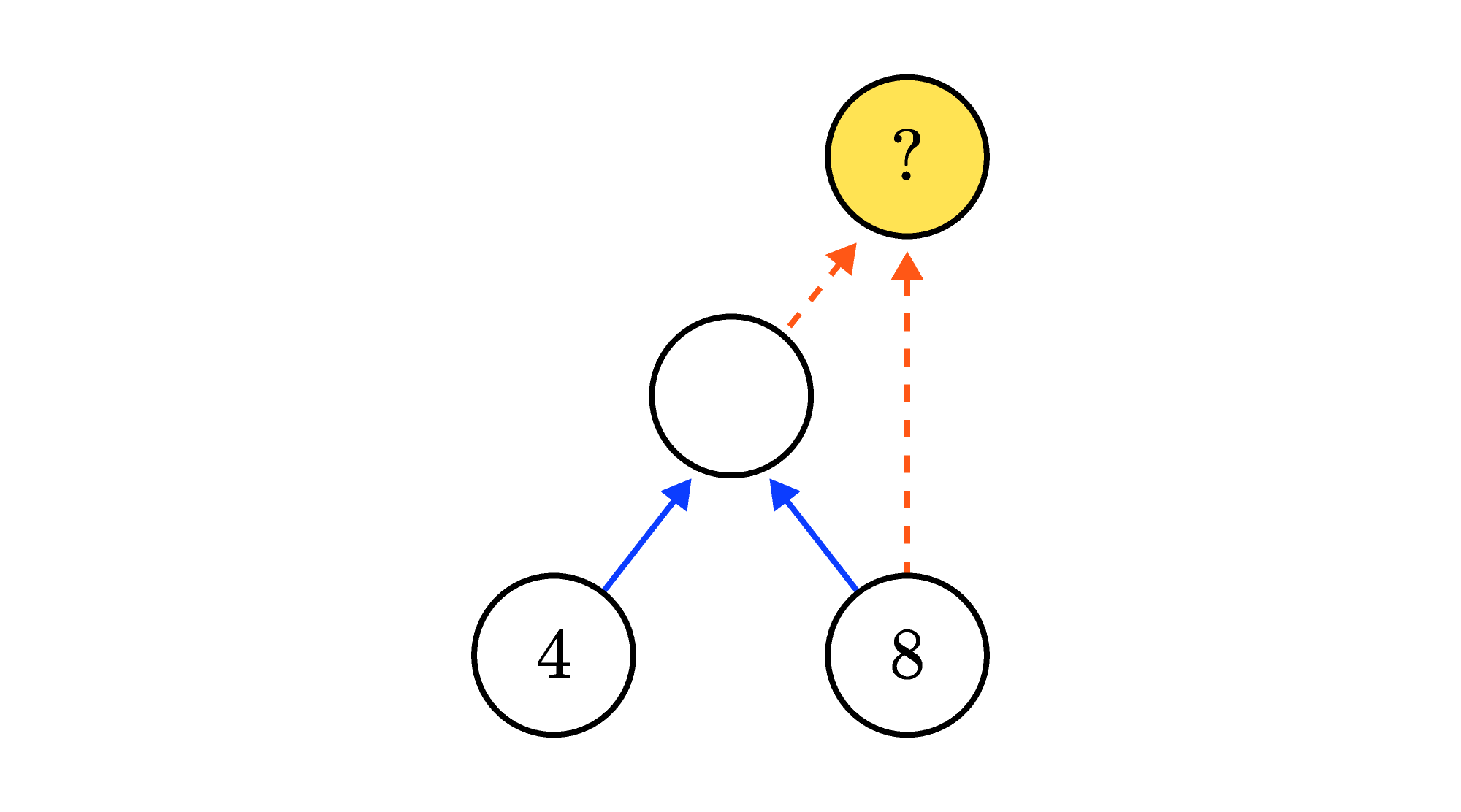The solution number is the sum of $8$ and another unknown number. We can find the unknown number since it's the product of $4$ and $8,$ which is $32.$ The solution must then be $32 + 8 = \mathbf{40}.$

On the other hand, some multi-step puzzles require us to work backwards through multiple steps.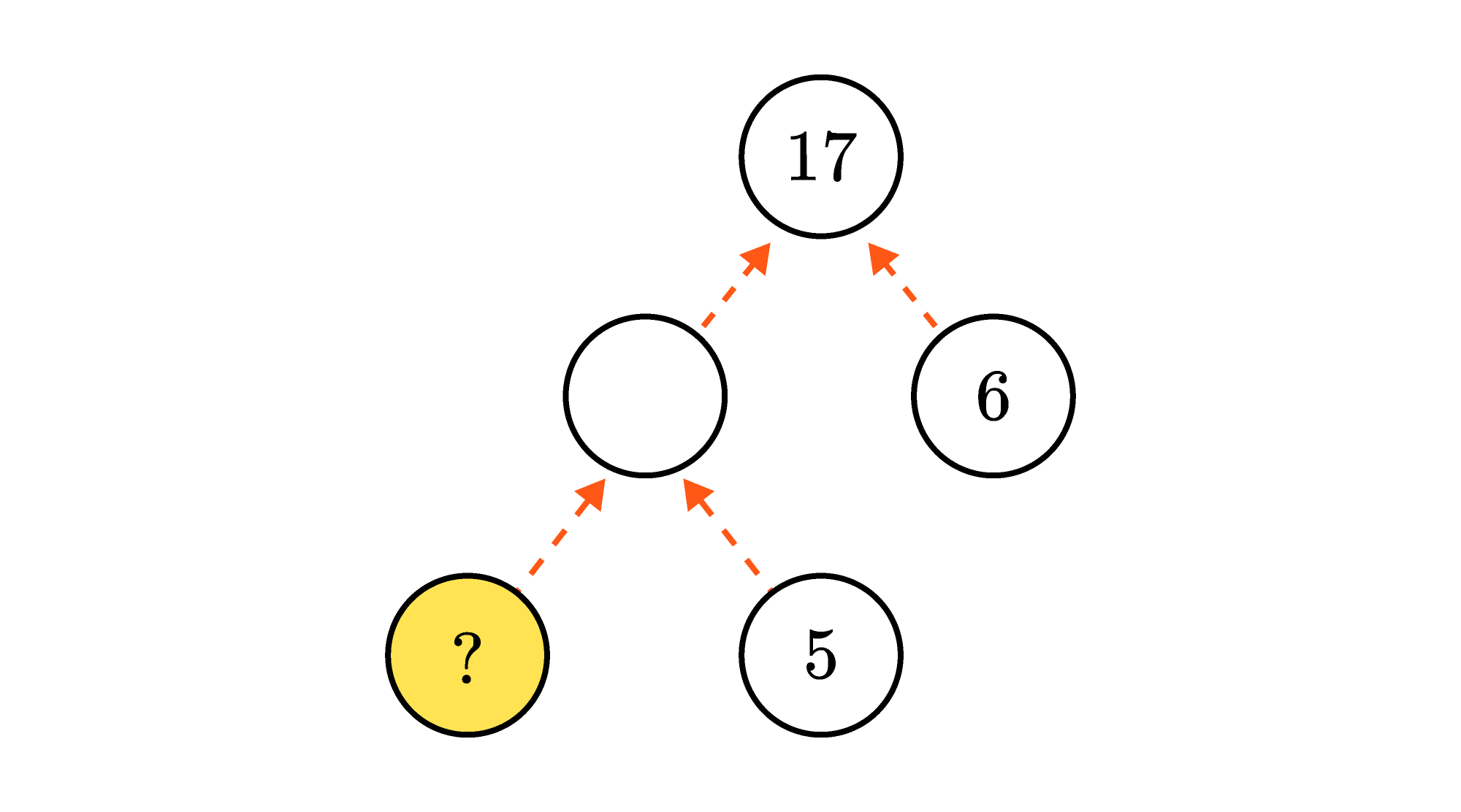Now, the solution number and $5$ add to some unknown number. We need to find that unknown number first. We can do so since it adds to $6$ to give us $17.$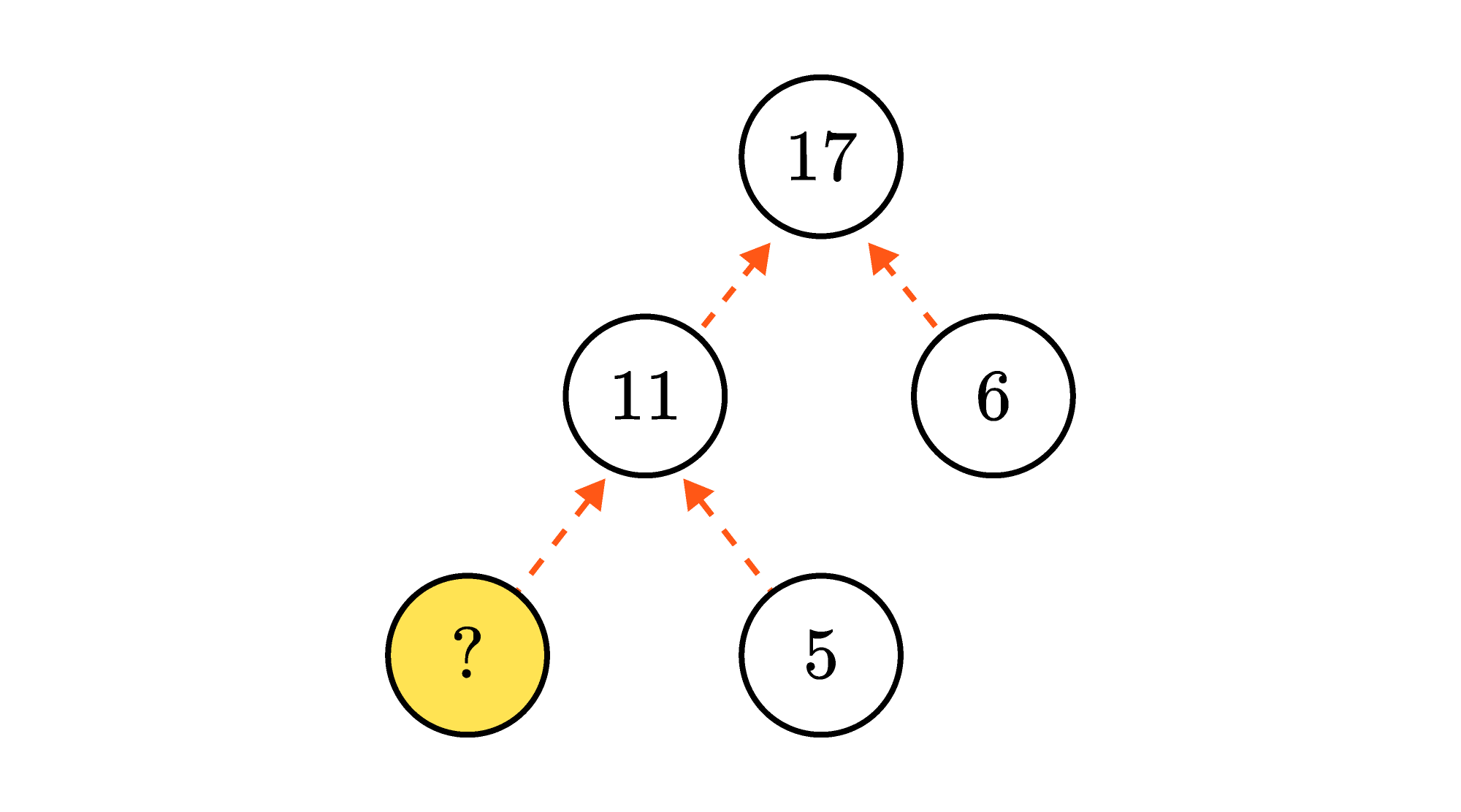The unknown number was $11,$ and it is the sum of our solution number and $5.$ This means our solution is $6.$

We can simplify some complex puzzles to make them easier to solve. In the puzzle below, $48$ is the product of three numbers: $2,$ the solution number, and some other unknown number.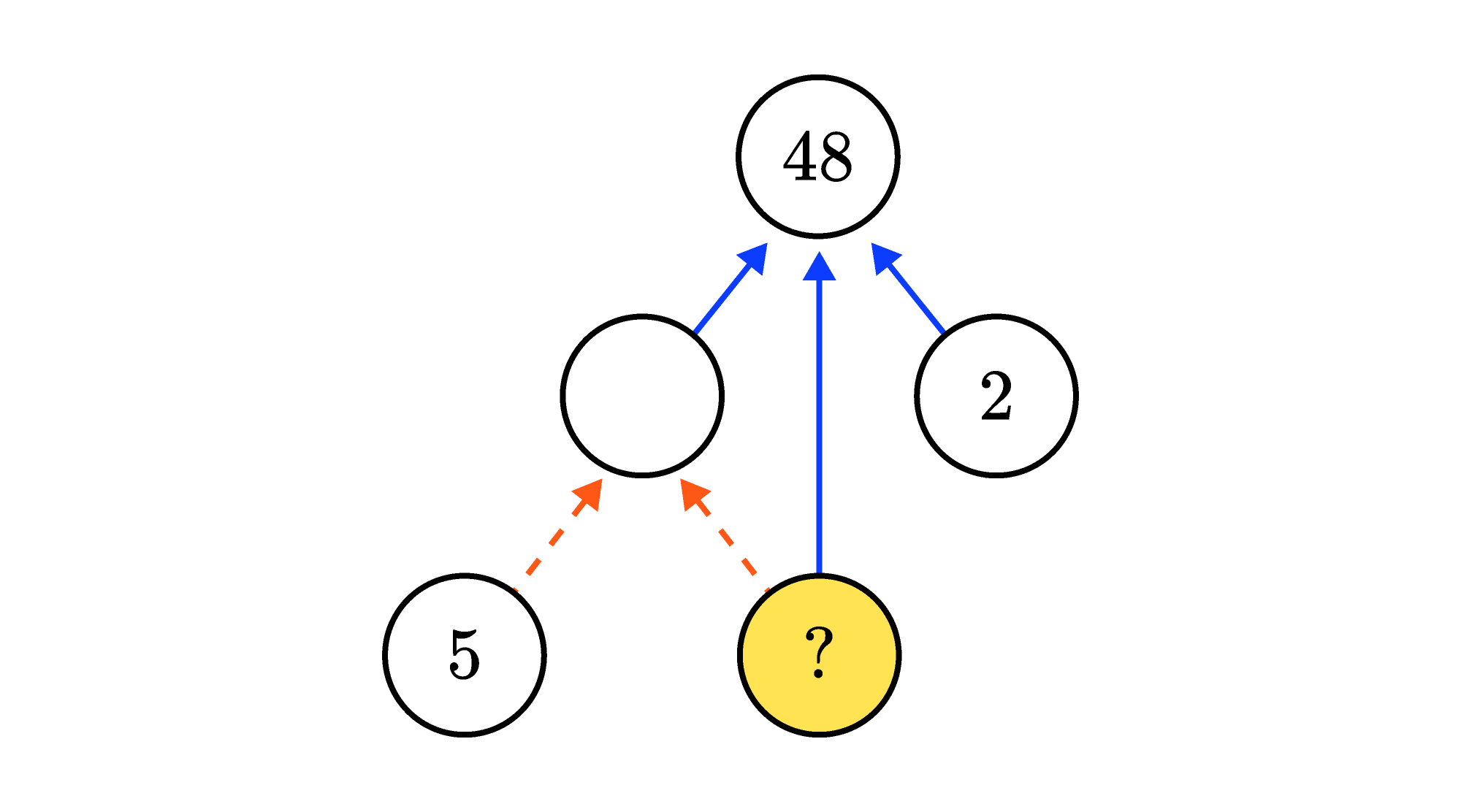Since $2 \times 24 = 48,$ the solution number and the other unknown number must multiply to $24.$ We can then get rid of the $2$ and show $24$ as a product of two numbers.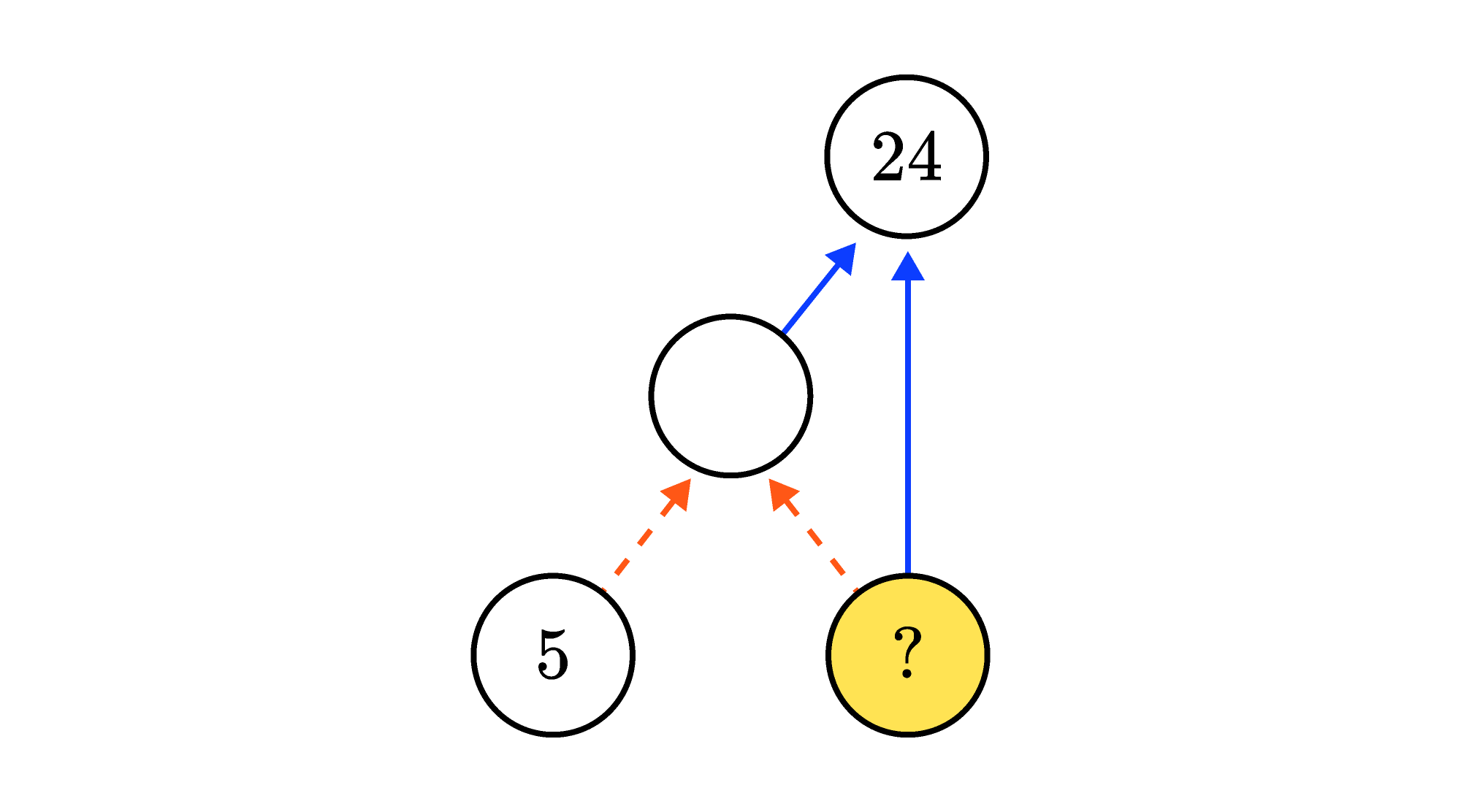The puzzle now tells us that $24$ is the product of two numbers: the solution number, and five added to the solution number. Let's list factor pairs of $24$ to find the solution:

\begin{aligned} 24 & \times 1 &&&&& (-24) \times (-1) \\ 12 & \times 2 &&&&& (-12) \times (-2) \\ 8 & \times 3 &&&&& (-8) \times (-3) \\ 6 & \times 4 &&&&& (-6) \times (-4) \\ \end{aligned}

There are two factor pairs whose numbers have a difference of $5.$ These pairs are $(8, 3)$ and $(-8, -3).$ This means there are two solutions: $3$ and $-8.$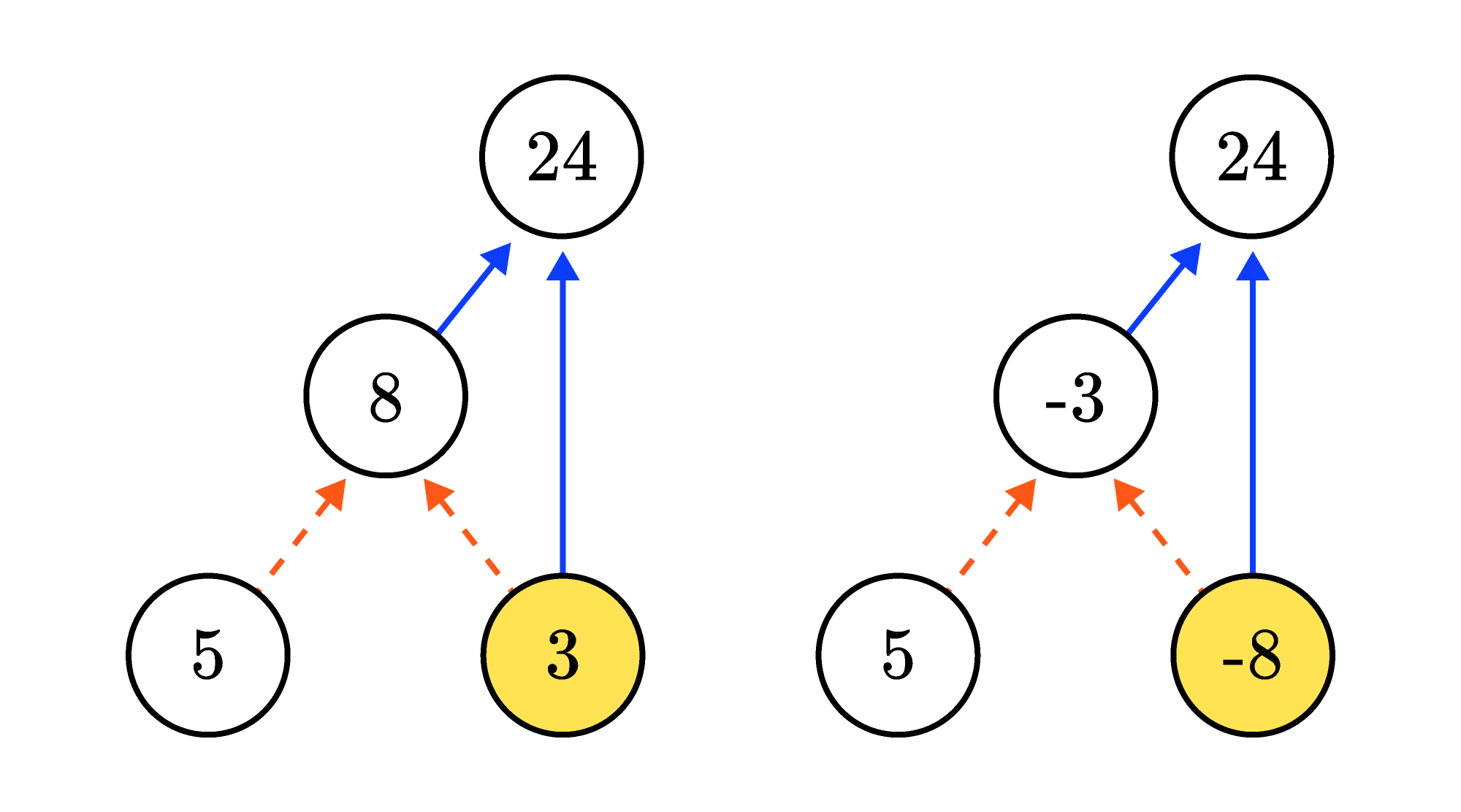Can you solve the circle puzzle below?

# Today's Challenge

In a circle puzzle like the one below, dashed arrows mean to add and solid arrows mean to multiply.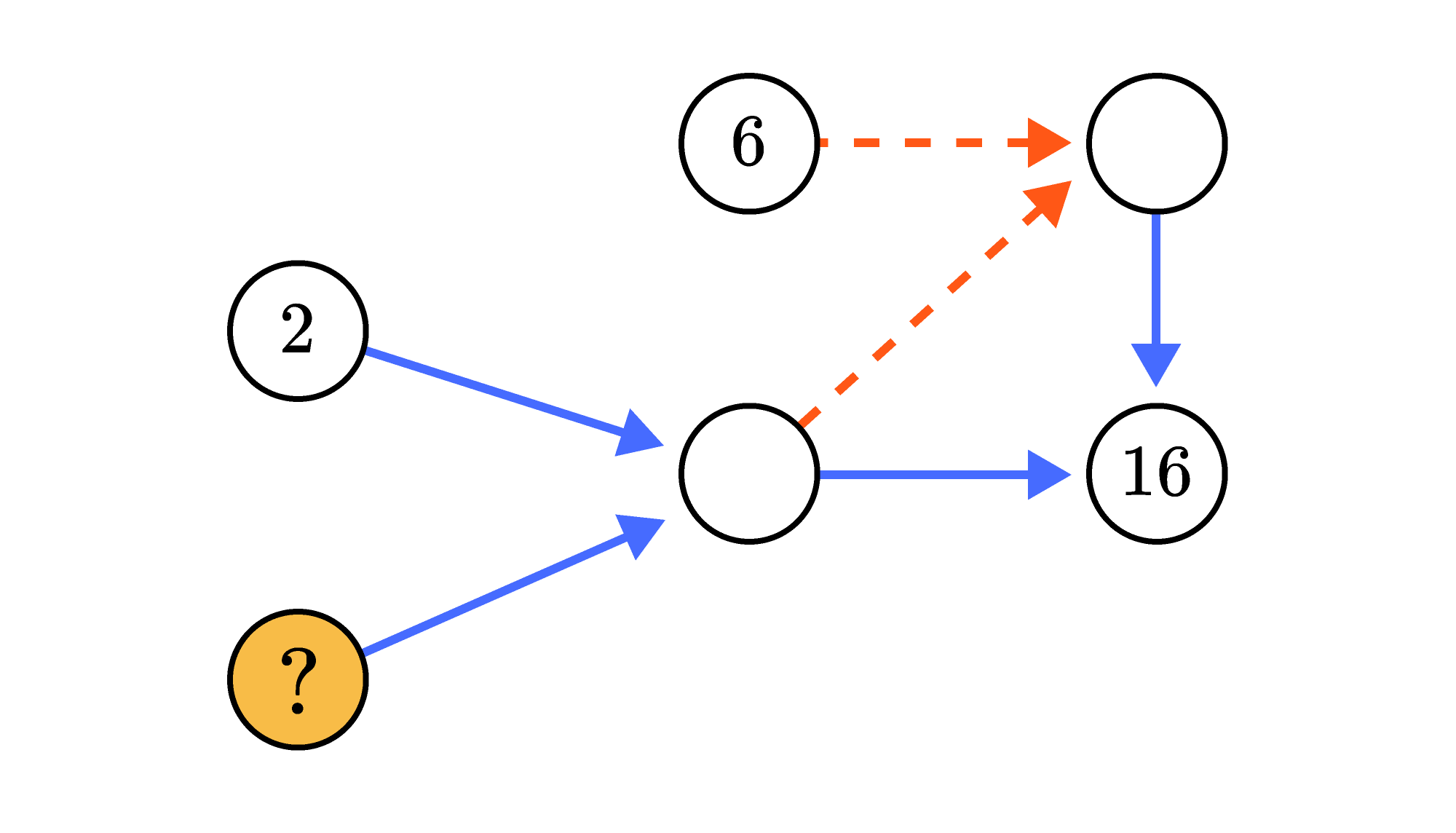What is the sum of all possible values for the place with the question mark?×